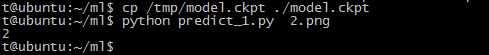机器学习-tensorflow 原

t@ubuntu:~\$ python
Python 2.7.6 (default, Oct 26 2016, 20:30:19)
[GCC 4.8.4] on linux2
>>> import tensorflow as tf
>>> hello=tf.constant('hello,tensorFlow!')
>>> sess = tf.Session()
>>> print sess.run(hello)
hello,tensorFlow!
>>> a = tf.constant(10)
>>> b = tf.constant(122)
>>> print sess.run(a+b)
132

# Copyright 2016 Niek Temme.
# Adapted form the on the MNIST biginners tutorial by Google.
#
# Licensed under the Apache License, Version 2.0 (the "License");
# you may not use this file except in compliance with the License.
# You may obtain a copy of the License at
#
#
# Unless required by applicable law or agreed to in writing, software
# distributed under the License is distributed on an "AS IS" BASIS,
# WITHOUT WARRANTIES OR CONDITIONS OF ANY KIND, either express or implied.
# See the License for the specific language governing permissions and
# limitations under the License.
# ==============================================================================

"""A very simple MNIST classifier.
Documentation at
http://niektemme.com/ @@to do

This script is based on the Tensoflow MNIST beginners tutorial
See extensive documentation for the tutorial at
https://www.tensorflow.org/versions/master/tutorials/mnist/beginners/index.html
"""

#import modules
import tensorflow as tf
from tensorflow.examples.tutorials.mnist import input_data

#import data
mnist = input_data.read_data_sets("MNIST_data/", one_hot=True)

# Create the model
x = tf.placeholder(tf.float32, [None, 784])
W = tf.Variable(tf.zeros([784, 10]))
b = tf.Variable(tf.zeros())
y = tf.nn.softmax(tf.matmul(x, W) + b)

# Define loss and optimizer
y_ = tf.placeholder(tf.float32, [None, 10])
cross_entropy = -tf.reduce_sum(y_*tf.log(y))

# init_op = tf.global_variables_initializer() 看版本，使用该行还是使用下面那行
init_op = tf.initialize_all_variables()
saver = tf.train.Saver()

# Train the model and save the model to disk as a model.ckpt file
# file is stored in the same directory as this python script is started
"""
The use of 'with tf.Session() as sess:' is taken from the Tensor flow documentation
on on saving and restoring variables.
https://www.tensorflow.org/versions/master/how_tos/variables/index.html
"""
with tf.Session() as sess:
sess.run(init_op)
for i in range(1000):
batch_xs, batch_ys = mnist.train.next_batch(100)
sess.run(train_step, feed_dict={x: batch_xs, y_: batch_ys})

save_path = saver.save(sess, "/tmp/model.ckpt")
print ("Model saved in file: ", save_path)

# Copyright 2016 Niek Temme.
#
# Licensed under the Apache License, Version 2.0 (the "License");
# you may not use this file except in compliance with the License.
# You may obtain a copy of the License at
#
#
# Unless required by applicable law or agreed to in writing, software
# distributed under the License is distributed on an "AS IS" BASIS,
# WITHOUT WARRANTIES OR CONDITIONS OF ANY KIND, either express or implied.
# See the License for the specific language governing permissions and
# limitations under the License.
# ==============================================================================

"""Predict a handwritten integer (MNIST beginners).

Script requires
1) saved model (model.ckpt file) in the same location as the script is run from.
(requried a model created in the MNIST beginners tutorial)
2) one argument (png file location of a handwritten integer)

Documentation at:
http://niektemme.com/ @@to do
"""

#import modules
import sys
import tensorflow as tf
from PIL import Image,ImageFilter

def predictint(imvalue):
"""
This function returns the predicted integer.
The imput is the pixel values from the imageprepare() function.
"""

# Define the model (same as when creating the model file)
x = tf.placeholder(tf.float32, [None, 784])
W = tf.Variable(tf.zeros([784, 10]))
b = tf.Variable(tf.zeros())
y = tf.nn.softmax(tf.matmul(x, W) + b)

init_op = tf.global_variables_initializer()
saver = tf.train.Saver()

"""
Load the model.ckpt file
file is stored in the same directory as this python script is started
Use the model to predict the integer. Integer is returend as list.

Based on the documentatoin at
https://www.tensorflow.org/versions/master/how_tos/variables/index.html
"""
with tf.Session() as sess:
sess.run(init_op)
saver.restore(sess, "/tmp/model.ckpt")
#print ("Model restored.")

prediction=tf.argmax(y,1)
return prediction.eval(feed_dict={x: [imvalue]}, session=sess)

def imageprepare(argv):
"""
This function returns the pixel values.
The imput is a png file location.
"""
im = Image.open(argv).convert('L')
width = float(im.size)
height = float(im.size)
newImage = Image.new('L', (28, 28), (255)) #creates white canvas of 28x28 pixels

if width > height: #check which dimension is bigger
#Width is bigger. Width becomes 20 pixels.
nheight = int(round((20.0/width*height),0)) #resize height according to ratio width
if (nheigth == 0): #rare case but minimum is 1 pixel
nheigth = 1
# resize and sharpen
img = im.resize((20,nheight), Image.ANTIALIAS).filter(ImageFilter.SHARPEN)
wtop = int(round(((28 - nheight)/2),0)) #caculate horizontal pozition
newImage.paste(img, (4, wtop)) #paste resized image on white canvas
else:
#Height is bigger. Heigth becomes 20 pixels.
nwidth = int(round((20.0/height*width),0)) #resize width according to ratio height
if (nwidth == 0): #rare case but minimum is 1 pixel
nwidth = 1
# resize and sharpen
img = im.resize((nwidth,20), Image.ANTIALIAS).filter(ImageFilter.SHARPEN)
wleft = int(round(((28 - nwidth)/2),0)) #caculate vertical pozition
newImage.paste(img, (wleft, 4)) #paste resized image on white canvas

#newImage.save("sample.png")

tv = list(newImage.getdata()) #get pixel values

#normalize pixels to 0 and 1. 0 is pure white, 1 is pure black.
tva = [ (255-x)*1.0/255.0 for x in tv]
return tva
#print(tva)

def main(argv):
"""
Main function.
"""
imvalue = imageprepare(argv)
predint = predictint(imvalue)
print (predint) #first value in list

if __name__ == "__main__":
main(sys.argv)TensorFlow 1.10.1 发布，谷歌开源机器学习库

2018/08/26
1K
4

oschina
2015/11/10
9.7K
19

2017/03/12
3.3K
5

TensorFlow 是 Google Brain 团队开发的强大的机器学习开源软件库，也是目前最流行的深度学习框架。可以说，要学机器学习和深度学习，就一定要掌握 TensorFlow。 TensorFlow 之所以受到如此欢...

Caicloud
2017/08/15
1
0

SZGDG
2018/09/12
18
0

shzwork

6
0
object 类中有哪些方法？

getClass(): 获取运行时类的对象 equals()：判断其他对象是否与此对象相等 hashcode()：返回该对象的哈希码值 toString()：返回该对象的字符串表示 clone()： 创建并返此对象的一个副本 wait...

happywe

6
0
Docker容器实战(七) - 容器中进程视野下的文件系统

JavaEdge

8
0

7
0

MtrS

5
0